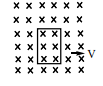A conducting square loop of side l and resistance R moves in its plane with a uniform velocity v perpendicular to one of its sides. A uniform and constant magnetic field B exists along the perpendicular to the plane of the loop as shown in figure. The current induced in the loop is

# A conducting square loop of side l and resistance R moves in its plane with a uniform velocity v perpendicular to one of its sides. A uniform and constant magnetic field B exists along the perpendicular to the plane of the loop as shown in figure. The current induced in the loop is1. A

Blv/R clock wise

2. B

Blv/R anticlock wise

3. C

2Blv/R anticlock wise

4. D

Zero

Register to Get Free Mock Test and Study Material

+91

Verify OTP Code (required)

### Solution:

As there is no change in magnetic flux with square, current induced is zero.

(or)

As the magnetic field is uniform and constant, there is no change in magnetic flux with square, current induced is zero.Register to Get Free Mock Test and Study Material

+91

Verify OTP Code (required)